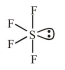# The molecular geometry of SF6 is octahedral.

Question:

The molecular geometry of $\mathrm{SF}_{6}$ is octahedral. What is the geometry of $\mathrm{SF}_{4}$ (including lone pair(s) of electrons, if any) ?

1. Trigonal bipyramidal

2. Square planar

3. Tetrahedral

4. Pyramidal

Correct Option: 1

Solution:$4 \sigma$ bonds $+1$ lone pair

$\therefore$ Shape (including lone pair of electrons) is

Trigonal bipyramidal